Nanomedicine, Volume I: Basic Capabilities

Robert A. Freitas Jr., Nanomedicine, Volume I: Basic Capabilities, Landes Bioscience, Georgetown, TX, 1999

10.3.1 Fluid Storage Tank Scaling

The ability of a vessel to store fluids at high pressure is determined by the rupture strength of the valve and pipe systems and by the tensile strength of the tank walls. A symmetric structural shell transmitting only normal stresses in orthogonal directions may employ either a stressed skin or a ribbed design. The stressed skin is more efficient because the same material carries stress in both directions and there is integral resistance to secondary torsional and bending loads.2023 For a stressed skin spherical pressure vessel of radius R with wall material of density rwall and working stress sw, containing fluid at pressure differential pfluid, the required wall thickness twall is given by:2023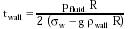{Eqn. 10.12}

where g = 9.81 m/sec2 (acceleration of gravity). For all but the most enormous macroscale tanks, the second term in the denominator is negligible and so the maximum pressure differential that can be restrained by a spherical microscale tank without bursting is: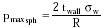{Eqn. 10.13}

Thus a tank of radius R = 1 micron made of diamondoid walls which are twall = 5 nm (~30 carbon atoms) thick with sw = 1010 N/m2 (~0.2 times the failure strength of diamond; Table 9.3) can store fluids up to a maximum pressure differential of pmaxsph = 1000 atm. Empty tank mass is Mtank ~ 4 p rwall twall R2 = (4 p rwall / 2 sw) pmaxsph R3, for twall << R. A spherical fullerene nanotank with defect-free single-carbon walls (twall ~ 0.34 nm thick), pressurized to pmaxsph ~ 1000 atm differential, must have R <~ 67 nm or it may burst.

Similarly, for a cylinder or pipe of radius R: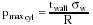{Eqn. 10.14}

Note that a hemispherical-capped cylindrical tank, pressurized until it explodes, will usually split its sides before blowing off its ends because pmaxsph = 2 pmax cyl. Wall stress for a cylindrical tube with Young's modulus E, inside radius R and wall thickness twall upon which is imposed a pressure differential of pcyl is ~ pcyl R / twall,362 giving a wall strain of S ~ pcyl R / twall E; hence a single-walled carbon nanotube with R = 20 nm, twall ~ 0.2 nm, and E ~ 1012 N/m2 pressurized to pcyl = 1000 atm stretches by S ~ 1%. (For comparison, aluminum soda pop cans are pressurized to ~2 atm differential, beer cans ~1 atm.)3703

For a toroid tank of small meridional radius r and large circumferential (hoop direction) radius R, the burst pressure in the meridional (pmax merid) and hoop (pmax hoop) directions are:2023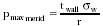{Eqn. 10.15}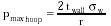{Eqn. 10.16}

Hence as pressure is raised, the toroidal tank fails first in the meridional direction, much like the capped cylinder.

Spherical pressure vessels are the most efficient. The maximum mass of gas that can be contained within a spherical tank at ideal gas pressures (Section 10.3.2) is Mgas ~ (4 p NA mgas / 3 Rgas Tgas) pmax R3, where NA = 6.023 x 1023 molecules/mole (Avogadro's number), mgas is the mass per gas molecule, Rgas = 8.31 J/mole-K (universal gas constant), and Tgas is gas temperature. Thus we observe that the ratio of gas mass to structural mass (Mgas/Mtank) at a given pressure is constant and independent of the size of the tank. This ratio reaches a maximum value at maximum pressurization; the maximum value is independent of both tank size and the maximum pressure selected (within the ideal gas range). In particular, Mgas/Mtank ~ 2 sw NA mgas / 3 Rgas Tgas rwall ~ 21 for nitrogen gas molecules (mgas = 4.65 x 10-26 kg/molecule) stored at temperature Tgas = 310 K. That is, the tank can store up to ~21 times its weight of gas.

Other important microtank design constraints include diffusion leakage and vessel flammability (Section 10.3.4), vapor pressure of tank materials at high temperatures (Section 10.3.5), thermal cycling during filling and discharging operations (Section 5.3.3), resistance to mechanical crushing, and susceptibility to acoustic resonances (e.g., the natural frequency of an rsph ~ 1 micron hollow diamondoid sphere is of order ~vsound / rsph ~ 20 GHz, fortunately much higher than most nanomedically useful frequencies).

Last updated on 24 February 2003